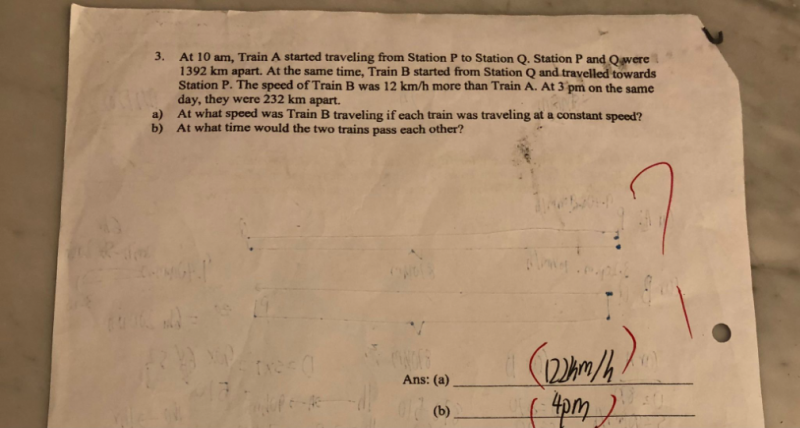# Question(a) Total distance PQ = 1392

Train A Speed = u            Train A Time = 5            Train A Distance travelled = 5u

Train B Speed = u+12      Train B Time = 5            Train B Distance travelled = 5(u+12) = 5u + 60

Distance apart = 232

Distance travelled by 2 trains in 5 hours = 1392-232 = 1160

5u + 5u + 60 = 1160

10u = 1160-60 = 110

u = 110

u+12 = 110+12 = 122

Hence Train B speed = 122 km/h

(b) Time taken to meet = t

110t + 122t = 1392

232t       = 1392

t         = 6

Hence starting from 10am, after 6 hours, it would be 4pm that they would pass each other.

0 Replies 2 Likes ✔Accepted Answer